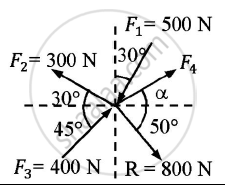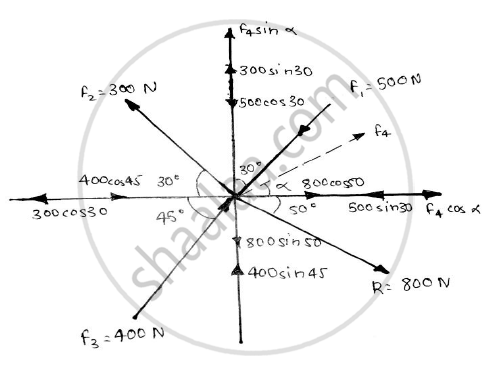# Find the force F4 , so as to give the resultant of the force as shown in the figure given below. - Engineering Mechanics

Short Note

Find the force F4 , so as to give the resultant of the force as shown in the figure given below.Given : Forces and their resultant
To find : Force F4

#### SolutionAssume that force F4 acts at an angle θ
Taking forces having direction towards right as positive and forces having direction upwards as positive.

Resolving forces along X direction :

-F_1sin30 – F_2cos30 + F_3cos45 + F_4cosθ = Rcos50

-500sin30 – 300cos30 + 400cos45 + F_4cosθ = 800cos50

F4cosθ = 741.195 …………(1)
Resolving forces along Y direction :

-F_1cos30 + F_2sin30 + F_3sin45 + F_4sinθ = -Rsin50  -500cos30 + 300sin30 + 400sin45 +F_4sin θ = -800sin50  F4sin θ = -612.6656 ……….(2)

Squaring and adding (1) and (2)

(F_4sinθ)^2 + (F_4cos θ)^2 = (-612.6656)^2 + (741.195)^2

F_4^2(sin^2 θ + cos^2 θ) = 924729.1173
F_4 = 961.6284 N

Dividing (2) by (1)

(F_4sinθ)/(F_4cosθ) = (−612.6656)/(741.195)

tan θ = -0.8266

θ = 39.5769o (in fourth quadrant)

F4 = 961.6284 N ( at an angle 39.5769o in fourth quadrant )

Concept: Resultant of concurrent forces
Is there an error in this question or solution?